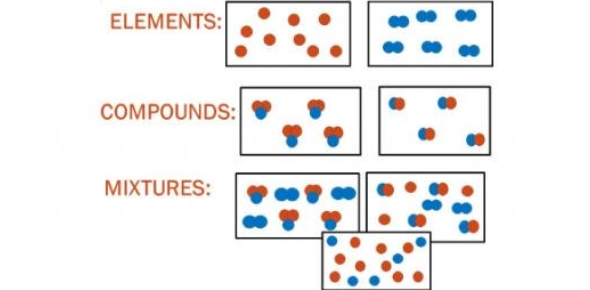# Chemistry- Mixtures, Elements & Compounds Quiz!

33 Questions | Total Attempts: 19179SettingsWe came to know the difference between a mixture, compound, and an element in chemistry class. A compound is a substance made up of two or more elements, whereas an element is a substance that cannot be split into simpler substances. Test out how much you remember about the topic by taking up the test below. All the best!

Questions and Answers
• 1.
An alloy is:
• A.

A compound

• B.

A solution of metals

• C.

A solution of gases

• 2.
• A.

Solute

• B.

Solution

• 3.
• A.

Solute

• B.

Solvent

• 4.
Na is the chemical symbol for:
• A.

Sodium

• B.

Tin

• C.

Nebraska

• 5.
C is the chemical symbol for:
• A.

Calcium

• B.

Carbon

• C.

Chlorine

• 6.
A mixture is two or more substances. It __________ be separated.
• A.

Can

• B.

Can not

• 7.
Are mixtures chemically combined?
• A.

Yes

• B.

No

• 8.
An Element is a simple pure substance. It __________ be separated?
• A.

Can

• B.

Can not

• 9.
A _______________ is two or more elements that are chemically combined.
• A.

Element

• B.

Compound

• C.

Mixture

• 10.
A compound __________ be separated?
• A.

Can

• B.

Can not

• 11.
Are compounds chemically combined?
• A.

Yes

• B.

No

• 12.
• A.

True

• B.

False

• 13.
An element can be broken down by physical or chemical means.
• A.

True

• B.

False

• 14.
Elements, compounds and mixtures are pure substances.
• A.

True

• B.

False

• 15.
Frying pans are usually made from non-metals because non-metals are good conductors of heat.
• A.

True

• B.

False

• 16.
Helium, which is put in balloons, is less dense than air.
• A.

True

• B.

False

• 17.
A compound has different properties than the elements that react to form it.
• A.

True

• B.

False

• 18.
Sugar water is an example of a heterogeneous mixture.
• A.

True

• B.

False

• 19.
Colloids are mixtures that will settle out.
• A.

True

• B.

False

• 20.
The chemical symbol for Mercury is HG.
• A.

True

• B.

False

• 21.
One example of changing a substance chemically is:
• A.

Filtering it

• B.

Burning it

• C.

Boiling it

• 22.
A person may put the following on his or her french-fries:
• A.

NaCl

• B.

Sn

• C.

HCl

• 23.
If a solute is unable to be dissolved in a given solvent, the solute is said to be:
• A.

Indissolveable

• B.

Soluble

• C.

Insoluble

• 24.
If a drink container tells you to "SHAKE WELL" before drinking, the mixture in the container is most likely a:
• A.

Solution

• B.

Suspension

• C.

Colloid

• 25.
A _________ ____________ is made of only one type of particle.
• A.

Pure substance

• B.

Compound

• C.

Solution

Related Topics